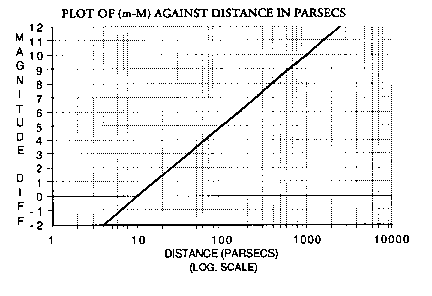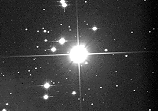# The Magnitude System

There are in fact two magnitude systems. One gives the brightness of a star as observed from here on Earth. This is the apparent magnitude . The other - absolute magnitude - gives a measure of the total amount of light being given out by the star in all directions. Both systems are logarithmic with a difference in magnitude of 5 being equivalent to a factor of 100 in brightness (NB small magnitudes correspond to bright stars). The absolute magnitude is directly related to the star's luminosity and the apparent magnitude can be measured here on Earth. From the Inverse Square Law , we can deduce an equation connecting the magnitudes and the star's distance. This allows us to calculate the distance in parsecs if we can find the star's apparent and absolute magnitudes (See graph).A bright star from a plate by the JKT (1m telescope on La Palma)